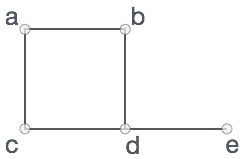# Python – Graphs

A graph is a pictorial representation of a set of objects where some pairs of objects are connected by links. The interconnected objects are represented by points termed as vertices, and the links that connect the vertices are called edges.
The various terms and functionalities associated with a graph is described in great detail in our tutorial here. In this chapter we are going to see how to create a graph and add various data elements to it using a python program. Following are the basic operations we perform on graphs.

• Display graph vertices
• Display graph edges
• Creating a graph

A graph can be easily presented using the python dictionary data types. We represent the vertices as the keys of the dictionary and the connection between the vertices also called edges as the values in the dictionary.

Take a look at the following graph −### In the above graph

```V = {a, b, c, d, e}
E = {ab, ac, bd, cd, de}```

We can present this graph in a python program as below.

```# Create the dictionary with graph elements
graph = { "a" : ["b","c"],
"b" : ["a", "d"],
"c" : ["a", "d"],
"d" : ["e"],
"e" : ["d"]
}

# Print the graph
print(graph)```

When the above code is executed, it produces the following result −

`{'c': ['a', 'd'], 'a': ['b', 'c'], 'e': ['d'], 'd': ['e'], 'b': ['a', 'd']}`

### Display graph vertices

To display the graph vertices we simple find the keys of the graph dictionary. We use the keys() method.

```class graph:
def __init__(self,gdict=None):
if gdict is None:
gdict = []
self.gdict = gdict

# Get the keys of the dictionary
def getVertices(self):
return list(self.gdict.keys())

# Create the dictionary with graph elements
graph_elements = { "a" : ["b","c"],
"b" : ["a", "d"],
"c" : ["a", "d"],
"d" : ["e"],
"e" : ["d"]
}

g = graph(graph_elements)

print(g.getVertices())
```

When the above code is executed, it produces the following result −

`['d', 'b', 'e', 'c', 'a']`

### Display graph edges

Finding the graph edges is little tricker than the vertices as we have to find each of the pairs of vertices which have an edge in between them.
So we create an empty list of edges then iterate thorugh the edge values associated with each of the vertices. A list is formed containing the
distinct group of edges found from the vertices.

```
class graph:

def __init__(self,gdict=None):
if gdict is None:
gdict = {}
self.gdict = gdict

def edges(self):
return self.findedges()
# Find the distinct list of edges

def findedges(self):
edgename = []
for vrtx in self.gdict:
for nxtvrtx in self.gdict[vrtx]:
if {nxtvrtx, vrtx} not in edgename:
edgename.append({vrtx, nxtvrtx})
return edgename

# Create the dictionary with graph elements
graph_elements = { "a" : ["b","c"],
"b" : ["a", "d"],
"c" : ["a", "d"],
"d" : ["e"],
"e" : ["d"]
}

g = graph(graph_elements)

print(g.edges())```

When the above code is executed, it produces the following result −

`[{'b', 'a'}, {'b', 'd'}, {'e', 'd'}, {'a', 'c'}, {'c', 'd'}]`

```class graph:

def __init__(self,gdict=None):
if gdict is None:
gdict = {}
self.gdict = gdict

def getVertices(self):
return list(self.gdict.keys())

# Add the vertex as a key
if vrtx not in self.gdict:
self.gdict[vrtx] = []

# Create the dictionary with graph elements
graph_elements = { "a" : ["b","c"],
"b" : ["a", "d"],
"c" : ["a", "d"],
"d" : ["e"],
"e" : ["d"]
}

g = graph(graph_elements)

print(g.getVertices())
```

When the above code is executed, it produces the following result −

`['f', 'e', 'b', 'a', 'c','d']`

Adding an edge to an existing graph involves treating the new vertex as a tuple and validating if the edge is already present.
If not then the edge is added.

```class graph:

def __init__(self,gdict=None):
if gdict is None:
gdict = {}
self.gdict = gdict

def edges(self):
return self.findedges()

edge = set(edge)
(vrtx1, vrtx2) = tuple(edge)
if vrtx1 in self.gdict:
self.gdict[vrtx1].append(vrtx2)
else:
self.gdict[vrtx1] = [vrtx2]

# List the edge names
def findedges(self):
edgename = []
for vrtx in self.gdict:
for nxtvrtx in self.gdict[vrtx]:
if {nxtvrtx, vrtx} not in edgename:
edgename.append({vrtx, nxtvrtx})
return edgename

# Create the dictionary with graph elements
graph_elements = { "a" : ["b","c"],
"b" : ["a", "d"],
"c" : ["a", "d"],
"d" : ["e"],
"e" : ["d"]
}

g = graph(graph_elements)
```[{'e', 'd'}, {'b', 'a'}, {'b', 'd'}, {'a', 'c'}, {'a', 'e'}, {'c', 'd'}]